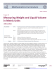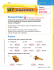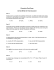# What is density? HS Chemistry POGIL Activity Why?

## Transcription

What is density? HS Chemistry POGIL Activity Why?
```HS Chemistry POGIL Activity
Topic: Density
What is density?
Why?
You’ve no doubt heard about density in science classes or in your everyday life. But what does it really
mean for one object to be more dense than another?
Model 1: Mass and Volume
A2
Material A
B2
A1
Material B
B1
1. How many dots are in the following cubes?
A1
A2
B1
B2
2. Based upon Model 1, what is mass?
3. What can you infer about how much mass each dot represents?
4. As volume increases on the graph’s X-Axis, what changes about each cube? What evidence
from Model 1 supports your thoughts?
5. Use the following data to calculate the volume for each of the cubes. Remember that the formula
for calculating volume for a cube is length x width x height.
Object
A1
A2
B1
B2
Length
2 cm
3.174 cm
2 cm
3.174 cm
Width
2 cm
3.174 cm
2 cm
3.174 cm
Height
2 cm
3.174 cm
2 cm
3.174 cm
Volume
6. According to your calculations, what would be the units for volume? Why?
8. What is similar about objects A1 and A2?
9. What is similar about objects B1 and B2?
10. Based upon your prior knowledge for density and the ideas you summarized in questions 8 and
9, create a definition for density with your group members.
Name: _____________________________
SUMMARIZING QUESTIONS
Instructions: Discuss these questions with your group. Write down your ideas. Leave space
to add additional ideas or thoughts you gain from the Summarizing Discussion.
1. Which of the following objects is more dense? Which is
less dense? Explain why
2. On the graph below, a line has been added to show the density of water. Based upon its density,
explain which objects you think will sink and which will float and why.
Water
3. Oil floats on top of water. Explain why and draw a picture (using dots like in Model 1) to show
why this is the case.
HS Chemistry POGIL Activity
Topics: Density
How can you calculate density?
Why?
How can you calculate density using the mass and volume of an object?
Model 2: Density Calculations
Object
Mass
Volume
Density
8 grams
5.33 mL
1.5 grams/mL
20 grams
13.33 mL
1.5 grams/mL
Object C
Object D
4. Evaluate Model 2. What is the same between Object C and Object D? Why is this (based on
their pictures?
5. How was density calculated using the mass and volume values?
6. Come up with a formula for calculating density (based on the numbers in Model 2).
7. Do you think that objects C and D are made out of the same or different material? Explain using
evidence from Model 2.
8. Complete the following table using data from the graph presented in Model 1, your volume
calculations in question 5, and your inferences from Model 2.
Object
Mass
Volume
Density
9. Calculate density for the following objects:
a. A 114 gram box that has the dimensions: length =3 cm, width = 3 cm, height = 7cm
b. A rock which has a mass of 60 grams and a volume of 20 mL
c. An unknown liquid with a mass of 10 grams and a volume of 15 mL
```

### Measuring Weight in the Metric System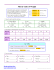### Stoichiometry Word Problems Name: You have been given 6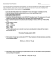### Quiz 5 Key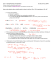### File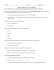### Stoichiometry Worksheet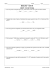### Room 21 News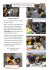### High-Fiber Power Pudding Recipe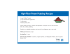### Document 6481104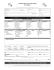### peaches and cream smoothie - Pancreatic Cancer Action Network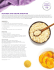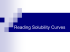### Gas Stoichiometry Worksheet Answer Key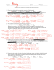### Dukono - Carlyle Collective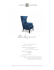### SWEET POTATOES DONUTS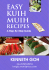### Winter Break 2014/2015 Pre AP Optional Home work Dimensional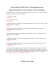### HONORS – THERMOCHEMISTRY INTRODUCTION WORKSHEET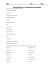### Sweets Sixteen - Persephone Bakery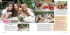### Document 6538338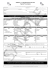### Document 6517586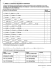### Day 5 âDensity & Buoyancy ___/64pts 2 ec pts printing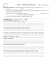### M in Measu n Me uring tric U g We Units ight a s and L